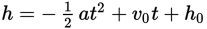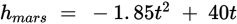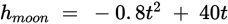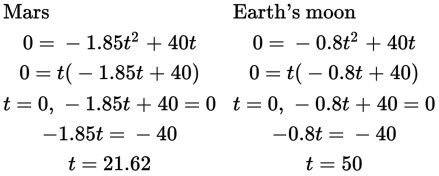# SAT Math Multiple Choice Question 936: Answer and Explanation

### Test Information

Question: 936

ObjectAcceleration Due to
Gravity (m/s2)
Earth9.8
Moon1.6
Mars3.7
Venus9.5
Jupiter24.5

4. The approximate height h of an object launched vertically upward after an elapsed time t is represented by the equation, where a is acceleration due to gravity, v0 is the object's initial velocity, and h0 is the object's initial height. The table above gives the acceleration due to gravityon Earth's moon and several planets. If two identical objects are launched verticallyupward at an initial velocity of 40 m/s from the surfaces of Mars and Earth's moon,approximately how many more seconds will it take for the moon projectile to returnto the ground than the Mars projectile?

• A. 21
• B. 28
• C. 36
• D. 50

Explanation:

B

Difficulty: Medium

Getting to the Answer: You know both projectiles begin at their respective surfaces and will be launched vertically at 40 m/s, so h0 = 0 and v0 = 40 for both. According to the table, acceleration due to gravity on Mars is 3.7 m/s2, so the final equation for the height of the Mars projectile is. Similarly, acceleration due to gravity on the moon is 1.6 m/s2, which makes the equation for the height of the moon projectile. Substitute 0 for h in each equation, and solve for t via factoring as follows: